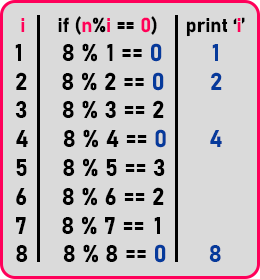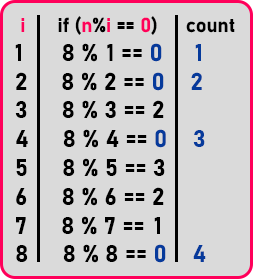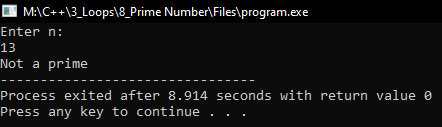# Prime Number using Loop in C++

## Prime Number using Loop in C++

In this article, I am going to discuss the Program to Check Whether a Given Number is Prime Number or Not using Loop in C++ with Examples. Please read our previous articles, where we discussed Perfect Number using Loop in C++ with Examples.

##### Prime Number:

A prime number is a number that is divisible by one and itself. No other number should divide it then only the number is a prime number. For example,

1. N = 8, factors are ‘1’, ‘2’, ‘4’ and ‘8’. Total 4 factors, so ‘8’ is not a prime number.
2. N = 13, factors are ‘1’ and ‘13’. Total 2 factors, so ‘13’ is a prime number.
3. N = 15, factors are ‘1’, ‘3’, ‘5’ and ‘15’. Total 4 factors, so ‘15’ is not a prime number.

Already we have discussed how to find factors of a number in previous articles. Below is the table for that,Whatever the number is given, we will start from 1, and up to that number we will check and if it is exactly divisible means the mod is ‘0, we will count them. If the count of the factors is ‘2’ then it will be a prime number otherwise it will not a prime number. For counting the factors, we will create a variable ‘count’ and initialize it with ‘0’ and we will modify the count variable when we found any factor.Now let us look at the program.

##### Program to check whether a given number is prime or not using loop in C++:
```#include <iostream>
using namespace std;
int main()
{
int n, count;
cout << "Enter n:" << endl;
cin >> n;

for (int i = 1; i <= n; i++)
{
if (n % i == 0)
{
count++;
}
}

if (count == 2)
cout << "Its a prime number";
else
cout << "Not a prime";

return 0;
}
```
###### Output:1.